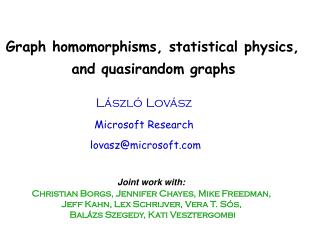# Graph homomorphisms, statistical physics, and quasirandom graphs - PowerPoint PPT PresentationDownload PresentationGraph homomorphisms, statistical physics, and quasirandom graphs

Graph homomorphisms, statistical physics, and quasirandom graphsDownload Presentation## Graph homomorphisms, statistical physics, and quasirandom graphs

- - - - - - - - - - - - - - - - - - - - - - - - - - - E N D - - - - - - - - - - - - - - - - - - - - - - - - - - -
##### Presentation Transcript

1. Graph homomorphisms, statistical physics, and quasirandom graphs László Lovász Microsoft Research lovasz@microsoft.com Joint work with: Christian Borgs, Jennifer Chayes, Mike Freedman, Jeff Kahn, Lex Schrijver, Vera T. Sós, Balázs Szegedy, Kati Vesztergombi

2. independent set coloring triangles Homomomorphism: adjacency-preserving map

3. Weighted version:

4. G connected 

5. L1966

6. probability that random map is a homomorphism every node in G weighted by 1/|V(G)| Homomorphism density: Homomorphism entropy:

7. if G has no loops Examples:

8. 1 1 2 1 1 H 3 3 -1 1/4 1/4 -1 -1 -1 -1 1/4 1/4 -1 3 3 H

9. atoms are in states (e.g. up or down): energy of interaction: energy of state: partition function: Hom functions and statistical physics interaction only between neighboring atoms: graph G

10. sparse G bounded degree partition function: denseG All weights in H are 1  hard-core model H=Kq, all weights are positive  soft-core model

11. : set of connected graphs Erdős – Lovász – Spencer Recall:

12. Kruskal-Katona Goodman 1 0 1/2 2/3 3/4 1 Bollobás Lovász-Simonovits

13. small probe (subgraph) small template (model) large graph

14. Kruskal-Katona Theorem for triangles: Turán’s Theorem for triangles: Erdős’s Theorem on quadrilaterals:

15. Connection matrix (for target graph G): Connection matrices k-labeled graph: k nodes labeled 1,...,k

16. ... k=2: ...

17. reflection positivity Main Lemma: is positive semidefinite has rank

18. k=2 Proof of Kruskal-Katona k=1

19. How much does the positive semidefinite property capture? ...almost everything!

20. reflection positivity equality holds in “generic” case (H has no automorphism) is positive semidefinite has rank Connection matrix of a parameter: graph parameter

21. ... finite sum is a commutative algebra with unit element Inner product: positive semidefinite suppose = k-labeled quantum graph:

22. Distance of graphs: Converse???

23. Example: Paley graphs p: prime 1 mod 4 Quasirandom graphs Thomason Chung – Graham – Wilson (Gn: n=1,2,...)is quasirandom, if d(Gn, G(n,p)) 0 a.s. How to see that these graphs are quasirandom?

24. For a sequence (Gn: n=1,2,...), the following are equivalent: (Gn) is quasirandom;  simple graph F; for F=K2 and C4. Converse if G’ is a random graph. Chung – Graham – Wilson

25. k=1: 1 p ... ... ... ... p p2 ... ... pk pk+1 Suppose that Want:

26. k=2: 1 p2 p2 p4 ... ... p2k p2k+2 ... ... ... ...

27. 1 pk ... ... ... ... pk p2k ... ... ... ... ... p|E(G’)| p|E(G)| ... ... ... ... k=deg(v)

28. density 0.2 0.1n 0.4n 0.2n 0.3n density 0.35 For a sequence (Gn: n=1,2,...), the following are equivalent: d(Gn, G(n,H))  0;  simple graph F; Generalized (quasi)random graphs 0.1 0.5 0.7 0.2 0.3 0.2 0.4 0.5 0.35 0.3

29. (Gn) left-convergent: (Gn) right-convergent: Recall:

30. Example: (C2n) is right-convergent But... (Cn) is not convergent for bipartite H

31. Any connection between left and right convergence?

32. (Gn) left-convergent: e.g. H=Kq, q>8D Graphs with bounded degree D